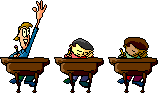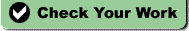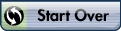# Chapter 3 Biochemistry Studyguide

### Javascript not enabled

 Name:Chapter 3 Biochemistry Study Guide

Modified True/False
Indicate whether the statement is true or false.  If false, change the identified word or phrase to make the statement true.
1.
Atoms have a positive charge. _________________________

2.
Electrons have very little mass. _________________________

3.
Molecules are atoms that have gained or lost one or more electrons. _________________________

4.
An atom with more electrons than protons has a positive charge. _________________________

5.
A covalent bond forms between two atoms that share electrons to form a molecule. _________________________

6.
Water molecules attract nonpolar molecules such as oil. _________________________

7.
The ability of water to retain heat helps cells maintain a constant internal temperature. _________________________

8.
The role of carbohydrates in cells is to supply energy. _________________________

9.
RNA is made up of a double strand of nucleotides. _________________________

10.
When cells break down food, the energy from the food is temporarily stored as ATP. _________________________

11.
The amount of energy needed to cause a chemical reaction to start is called activation energy. _________________________

12.
Without enzymes, chemical reactions necessary for life would not occur quickly or easily enough to sustain life. _________________________

13.
When an enzyme binds with its substrate, the activation energy needed for the chemical reaction to occur is increased. _________________________

Multiple Choice
Identify the choice that best completes the statement or answers the question.
14.
Atoms are not composed of
 a. protons. c. electrons. b. neutrons. d. chemical bonds
15.
The electrons of an atom
 a. are found in the nucleus along with the protons. b. occupy the space surrounding the nucleus. c. have a positive charge. d. are attached to the positive charge of neutrons.
16.
The smallest particle of matter that can retain the chemical properties of carbon is a carbon
 a. molecule. c. atom. b. macromolecule. d. element.
17.
A substance that is composed of only one type of atom is called a(n)
 a. compound. c. element. b. cell. d. molecule.
18.
All matter is composed of
 a. cells. c. atoms. b. molecules. d. carbon.
19.
Covalent bonds form when
 a. two molecules of water share electrons. b. a molecule of water becomes an ion. c. two hydrogen atoms share electrons with one oxygen atom. d. two hydronium ions are attracted to each other.
20.
Ionic bonds form between particles that have
 a. opposite charges. c. no charges. b. the same charge. d. neutral charges.
21.
The bond formed when two atoms share a pair of electrons is called a(n)
 a. hydrogen bond. c. covalent bond. b. ionic bond. d. water bond.
22.
Sharing of electrons in the outer energy levels of two atoms
 a. results in ion formation. b. makes both atoms stable. c. only occurs if both are atoms of the same element. d. is found only among carbon atoms.
23.
A molecule that has a partial positive charge on one side and a partial negative charge on the other side is called a(n)
 a. nonpolar molecule. c. charged molecule. b. polar molecule. d. ion.
24.
Nonpolar molecules have
 a. no negative or positive ends. c. only a negative end. b. both negative and positive ends. d. only a positive end.
25.
When placed in the same container, oil and water do not mix because
 a. they are both polar. c. they are both nonpolar. b. water is polar and oil is nonpolar. d. water is nonpolar and oil is polar.
26.
Attractions between water molecules are called
 a. covalent bonds. c. polar bonds. b. ionic bonds. d. hydrogen bonds.
27.
Water is a polar molecule because
 a. it contains two hydrogen atoms for each oxygen atom. b. it has a charge. c. different parts of the molecule have slightly different charges. d. it does not have a charge.
28.
Which is not a unique property of water?
 a. Frozen water floats. b. Water retains a large amount of heat. c. Water molecules stick to each other. d. Water cools rapidly.
29.
Acidic solutions have a pH that is
 a. less than 7. c. a negative number. b. between 7 and 14. d. more than 7.
30.
Hydronium ions, H+, react with hydroxide ions, OH-, to form
 a. water. c. a base. b. an acid. d. a buffer.
31.
Which of the following is not a large biomolecule?
 a. carbohydrate c. lipid b. ice d. nucleic acid
32.
The building blocks of most biomolecules contain the element
 a. carbon. c. calcium. b. nitrogen. d. sodium.
33.
Which of the following molecules are classified as carbohydrates?
 a. amino acids c. nucleotides b. fats d. sugars
34.
Polysaccharides are
 a. carbohydrates. c. proteins. b. lipids. d. nucleic acids.Molecule A Molecule B
35.
Refer to the illustration above. Molecule A is a
 a. carbohydrate. c. nucleic acid. b. lipid. d. protein.
36.
Plants store glucose in the form of
 a. starch. c. cellulose. b. glycogen. d. chitin.
37.
Which of the following is a carbohydrate?
 a. ATP c. wax b. steroid d. sucrose
38.
Lipids are
 a. soluble in water. b. made of chains of amino acids. c. linked together with peptide bonds. d. used by the body for storing energy.
39.
All of the following are examples of lipids except
 a. oil. c. steroids. b. starch. d. candle wax.
40.
Which molecules are most closely related to lipids?
 a. amino acids b. long chains of carbon and hydrogen c. nucleotides d. sugars
41.
Which of the following molecules are most closely related to proteins?
 a. amino acids c. nucleotides b. monosaccharides d. sugars
42.
Long chains of amino acids are found in
 a. carbohydrates. c. proteins. b. lipids. d. sugars.
43.
The overall shape of a single chain of amino acids in a protein is that protein’s
 a. primary structure. c. tertiary structure. b. secondary structure. d. quaternary structure.
44.
The amino group of one amino acid is linked to the carboxyl group of another amino acid in a protein. This link is a(n)
 a. peptide bond. c. ionic bond. b. hydrogen bond. d. polar bond.
45.
What gives each amino acid its unique properties?
 a. its side group c. its carboxyl group b. its amino group d. its sequence
46.
The two types of nucleic acids are
 a. RNA and ATP. c. DNA and ATP. b. DNA and RNA. d. nucleotides and ATP.
47.
Which of the following molecules are most closely related to nucleic acids?
 a. amino acids c. nucleotides b. fats d. sugars
48.
DNA stores
 a. fat. c. energy. b. carbohydrates. d. hereditary information.
49.
The two strands of a DNA molecule are held together by
 a. ionic bonds. c. hydrogen bonds. b. covalent bonds. d. peptide bonds.
50.
Which of the following is not part of a nucleotide in a DNA molecule?
 a. three phosphate groups c. a base b. a sugar d. a phosphate group
51.
ATP (adenosine triphosphate) stores energy in the bonds between its
 a. hydrogen atoms. c. nucleotides. b. carbon atoms. d. phosphate groups.
52.
When paper burns, the paper combines with oxygen to form carbon dioxide and water vapor. How does the mass of carbon dioxide plus water vapor compare with the mass of the paper plus oxygen?
 a. The mass of carbon dioxide plus water vapor is greater than that of paper plus oxygen. b. The mass of carbon dioxide plus water vapor is less than that of paper plus oxygen. c. It is impossible to tell. d. The mass of carbon dioxide plus water vapor is identical to that of paper plus oxygen.
53.
The law of conservation of energy states that energy cannot be
 a. changed from one form to another. b. taken from the surroundings in a reaction. c. released into the surroundings in a reaction. d. created or destroyed in a chemical reaction.
54.
A chemical reaction will probably occur between two substrate molecules if they
 a. are far apart. b. are aligned in the wrong spatial orientation. c. have large amounts of kinetic energy. d. have little kinetic energy.
55.
Reducing activation energy
 a. is a violation of the laws of nature. b. requires higher temperatures than those found within cells. c. occurs only when reactants are quickly added to the reaction mixture. d. is accomplished by the action of enzymes on reactants.56.
Refer to the illustration above. Which of the following statements regarding the graph is true?
 a. Reaction 2 occurs faster than Reaction 3 because Reaction 2 requires more energy than Reaction 3. b. The difference between the graphs shown for Reaction 2 and Reaction 3 occurs because of a difference in the activation energy of these reactions. c. Reactant A contains more energy at the beginning of the reaction than product C has at the end of the reaction. d. Product B contains more energy at the end of the reaction than reactant A has at the beginning of the reaction.
57.
Refer to the illustration above. Reaction 3 in the graph
 a. probably occurred in the presence of an enzyme. b. requires more activation energy than Reaction 2. c. is the same as Reaction 1, but faster. d. is slower than Reaction 2.
58.
The molecule on which an enzyme acts is called a(n)
 a. active site. c. polar molecule. b. inactive site. d. substrate.
59.
An enzyme
 a. is used up in a reaction. b. raises the activation energy of a reaction. c. bonds with an active site on a substrate molecule. d. lowers the activation energy of a reaction.
60.
Enzymes
 a. are able to heat up molecules so that they can react. b. always provide carbon dioxide for chemical reactions. c. hold substrate molecules in the correct alignment. d. absorb excess heat so that reactions occur at low temperatures.
61.
A cell contains
 a. thousands of different kinds of enzymes, each assisting in a different chemical reaction. b. one kind of enzyme that assists in thousands of different chemical reactions. c. approximately 100 kinds of enzymes, each assisting in a different chemical reaction. d. one enzyme that assists in reactions involving protein substrates and one enzyme that assists in reactions involving substrates that are not proteins.

Completion
Complete each statement.
62.
The negatively charged particles in an atom are ____________________.

63.
Protons and neutrons are found in the ____________________ of an atom.

64.
A(n) ____________________ is a group of atoms held together by covalent bonds.

65.
Sodium chloride, NaCl, is an example of a(n) _________________.

66.
A(n) ____________________ bond forms between two atoms sharing electrons.

67.
The attraction between oppositely charged ions results in the formation of a(n) ____________________.

68.
Weak chemical attractions between polar molecules are ____________________ bonds.

69.
Lipids are ____________________ molecules because they have no negative and positive poles.

70.
Water heats and cools ____________________.

71.
The attraction that causes water and other liquids to form drops and thin films is ____________________.

72.
The medium in which most cellular events take place is ____________________.

73.
Substances with a pH less than 7 are ____________________.

74.
Substances with a pH greater than 7 are ____________________.

75.
The pH scale measures the concentration of ____________________ ions in solutions.

76.
A carbon atom can bond with four other atoms because it has ____________________ valence electrons.

77.
A(n) ____________________ contains carbon, hydrogen, and oxygen in a ratio of 1:2:1.

78.
Amino acids are the building blocks of __________________.

79.
Most proteins consist of two or more ________________ that twist and fold around each other.

80.
Long chains of nucleotides are called ____________________.

81.
The ability to move or change matter is ____________________.

82.
All living things require a source of ____________________ to carry out their life activities.

83.
The starting materials for chemical reactions are called ____________________, while the new substances that are formed are called ____________________.

84.
The energy needed to start a chemical reaction is called ____________________.

85.
A biochemical reaction can be speeded up by adding a(n) ____________________, which lowers the amount of activation energy required to start the reaction.

86.
The portion of an enzyme molecule into which a specific substrate can fit is called the ____________________.

87.
How many different kinds of atoms are there in an element?

88.
How does an atom become an ion?

89.
An ionic bond forms between what two kinds of particles?

90.
What makes a water molecule polar?

91.
How well do nonpolar molecules dissolve in water?

92.
What is an attraction between particles of different substances called?

93.
What are the two most common elements found in large biomolecules?

94.
What is the function of lipids in cells?

95.
What determines the primary structure of a protein?

96.
Where do your cells get most of the energy they need for metabolism?

97.
How do enzymes speed up a chemical reaction?

Problem
98.

The following statements are about the molecule ATP (adenosine triphosphate). For each statement, first determine whether it is true or false. Then, if it is false, rewrite the statement so that it is correct.

 a. ATP is chemically similar to a carbohydrate. b. Cells require ATP to function. c. None of the energy in food molecules is stored in ATP. d. ATP is the primary source of energy for chemical reactions occurring in all cells of all living organisms.99.

Refer to the illustration above. The graph depicts the relative energy levels of the products and reactants for the following chemical reaction: A + B « C + D.

 a. Which substances, A, B, C, and/or D, are present at point 1 on the graph? b. Which substances, A, B, C, and/or D, are present at point 3 on the graph? c. Why is point 2 at a higher energy level than point 1? d. Why is point 3 at a lower energy level than point 1? e. Draw a dashed line on the graph indicating how the energy level of this reaction over time would be different if the enzyme that catalyzes the reaction were not present.

Enzymes catalyze a variety of biological reactions. The extent to which they can speed up the rate of a reaction is dependent on a number of factors. Draw a graph for the following condition(s), showing the effect of altering one factor on the relative rate of a reaction. Use “0” to indicate no activity, and “1” through “10” to indicate increasing rate of reaction from very low to very high. Include a label for your graph(s).
100.
Refer to the paragraph above, and then draw your graph in the space below. Enzyme #1 is inactive between 0°C and 10°C. It is active above 10°C, with the rate increasing steadily until the temperature reaches 40°C. At 40°C, the rate of the reaction is very high. Above 40°C, the rate decreases steadily until the temperature reaches 60°C, at which point the enzyme is no longer active.

101.
Refer to the paragraph above, and draw your graph. Enzyme #2 is inactive at a pH of 1–4. It is active above a pH of 4, with the rate increasing slowly until the pH reaches 6. Above a pH of 6, the rate increases sharply until the pH reaches 7. Above a pH of 7, the rate decreases sharply until the pH reaches 8. Above a pH of 8, the rate decreases gradually until the pH reaches 10, at which point the enzyme is no longer active.

102.
Refer to the paragraph above, and draw your graph. Enzyme #3 is inactive when the concentration of the substrate it acts upon is zero. The enzyme is active when the substrate concentration is above zero, with the rate increasing only very slightly until the concentration reaches 30%. Above 30%, the rate increases very sharply to a high level, until the concentration reaches 60%. Above a concentration of 60%, the rate increases very little. The rate does not increase when the substrate concentration is above 80%.

Essay
103.
Describe how an enzyme can function in speeding up a chemical reaction within a cell.